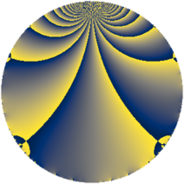Properties

 Label 639.1.dLevel $639$ Weight $1$ Character orbit 639.d Rep. character $\chi_{639}(496,\cdot)$ Character field $\Q$ Dimension $3$ Newform subspaces $1$ Sturm bound $72$ Trace bound $0$

Related objects

Defining parameters

 Level: $$N$$ $$=$$ $$639 = 3^{2} \cdot 71$$ Weight: $$k$$ $$=$$ $$1$$ Character orbit: $$[\chi]$$ $$=$$ 639.d (of order $$2$$ and degree $$1$$) Character conductor: $$\operatorname{cond}(\chi)$$ $$=$$ $$71$$ Character field: $$\Q$$ Newform subspaces: $$1$$ Sturm bound: $$72$$ Trace bound: $$0$$

Dimensions

The following table gives the dimensions of various subspaces of $$M_{1}(639, [\chi])$$.

Total New Old
Modular forms 16 4 12
Cusp forms 12 3 9
Eisenstein series 4 1 3

The following table gives the dimensions of subspaces with specified projective image type.

$$D_n$$ $$A_4$$ $$S_4$$ $$A_5$$
Dimension 3 0 0 0

Trace form

 $$3 q + q^{2} + 2 q^{4} + q^{5} + 2 q^{8} + O(q^{10})$$ $$3 q + q^{2} + 2 q^{4} + q^{5} + 2 q^{8} - 2 q^{10} + q^{16} - q^{19} - 4 q^{20} + 2 q^{25} + q^{29} + 3 q^{32} - q^{37} - 5 q^{38} - 4 q^{40} - q^{43} + 3 q^{49} + 3 q^{50} - 2 q^{58} - 3 q^{71} - q^{73} - 5 q^{74} - 3 q^{76} - q^{79} - 2 q^{80} + q^{83} + 2 q^{86} + q^{89} + 2 q^{95} + q^{98} + O(q^{100})$$

Decomposition of $$S_{1}^{\mathrm{new}}(639, [\chi])$$ into newform subspaces

Label Dim. $$A$$ Field Image CM RM Traces $q$-expansion
$a_{2}$ $a_{3}$ $a_{5}$ $a_{7}$
639.1.d.a $3$ $0.319$ $$\Q(\zeta_{14})^+$$ $D_{7}$ $$\Q(\sqrt{-71})$$ None $$1$$ $$0$$ $$1$$ $$0$$ $$q+\beta _{1}q^{2}+(1+\beta _{2})q^{4}-\beta _{2}q^{5}+(1+\beta _{2})q^{8}+\cdots$$

Decomposition of $$S_{1}^{\mathrm{old}}(639, [\chi])$$ into lower level spaces

$$S_{1}^{\mathrm{old}}(639, [\chi]) \cong$$ $$S_{1}^{\mathrm{new}}(71, [\chi])$$$$^{\oplus 3}$$